# Division + fractions - math problems

#### Number of problems found: 235

• If Ben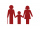If Ben is ⅕ the age of his father, ¼ the age of his mother, and twice the age of his sister, how old are his parents and sister if he is 8 years old?
• A sumA sum of money is shared between Peter, John and Henry in the ratio 2:3:5. a) express Henry's share as fraction of John's share. b) what fraction of the whole sum of money is John's share?
• Benhur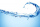Benhur boiled 1 1/4 liters of water in a kettle. After 10 1/2 minutes he measured the water again. It had 3/4 liters left in the kettle. What is the amount of water that evaporates every minutes?
• Sir ToffieOne hot afternoon, Sir Toffie went to his backyard to plant monggo. In his seed box, he found out that different seeds like monggo, pechay, eggplant, and tomatoes were accidentally mixed because of the strong wind. He sieved the mixed seeds and was able t
• Jose studiedJose studied for 4 and 1/2 hours on Saturday and another 6 and 1/4 hours on Sunday. How many subjects did he study if he has alloted 1 and 1/2 hours per subject on Saturday and 1 and 1/4 hours per subject on Sunday?
• In dividingIn dividing fractions, get the reciprocal of the divisor and change division symbol to multiplication symbol. 2/3 : 5/6
• One half 2One half pizza will be divide among 3 pupils. Each pupil receive 1/6. Is it true or false?
• Pie division5/8 of a pie divide 6 pieces. Each friend got 1/6.  What fraction  of the whole pie does each person receive?
• How many 17How many fifths are there in four wholes? How many 1/5 are there in 4?
• Aspect ratioDetermine the ratio of the length to the width of a picture frame that is 12 3/4 inches long and 8 1/2 inches wide.
• Quotient and divisionFind the quotient of 3/4 and 1/4.
• Simplify expression with mixedWhen (7¹/3 + 2¾)÷(2 + 2¼ × 1¹/3) is simplified the result is?
• Quotient and divisionWhat is the quotient of 2/9 and 1/8?
• A PVC pipeA PVC pipe 12 feet long is to be cut into 1 1/2 foot pieces.  How many pieces can a plumber get out of this length pipe?
• Simplest form 3What fraction is 15 of 35 in simplest form?
• Licorice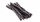A piece of licorice is 2/3 yard long.  If it is cut into 10 equal pieces, how long will each piece of licorice be?
• Divide 13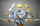Divide. Simplify your answer and write as an improper fraction or whole number. 14÷8/3
• The rope 2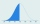The rope in the school gym is 10 ½ feet long. To make it easier to climb, Coach Hill tied a knot in the rope every ¾ foot. How many knots are in the rope?
• How many 16How many three-tenths are there in two and one-fourths?
• Fraction expressionWhich expression is equivalent to : minus 9 minus left parenthesis minus 4 start fraction 1 divided by 3 end fraction right parenthesis

Do you have an exciting math question or word problem that you can't solve? Ask a question or post a math problem, and we can try to solve it.

We will send a solution to your e-mail address. Solved examples are also published here. Please enter the e-mail correctly and check whether you don't have a full mailbox.

Need help to calculate sum, simplify or multiply fractions? Try our fraction calculator. Division Problems. Fraction Word Problems.